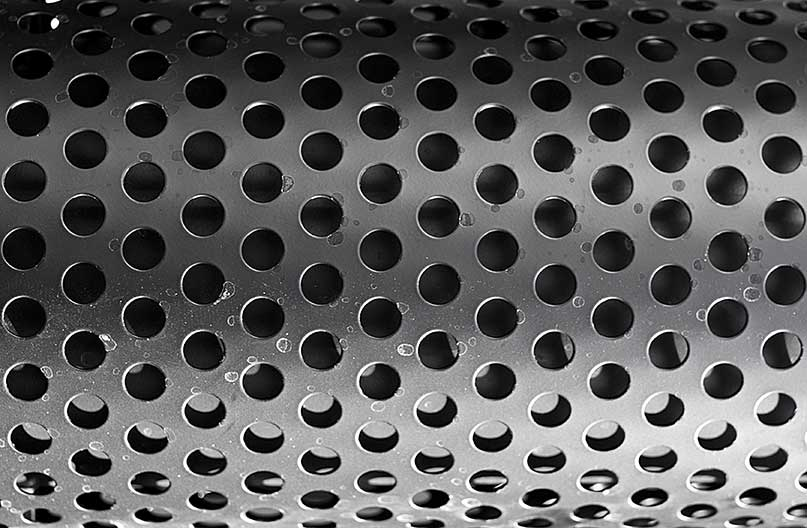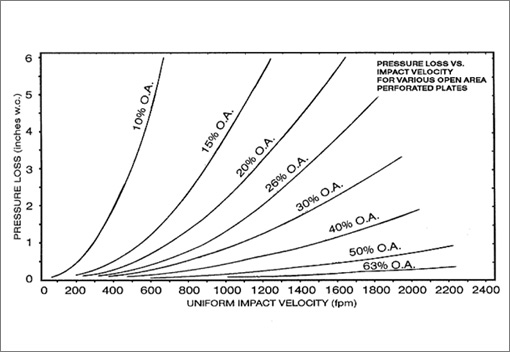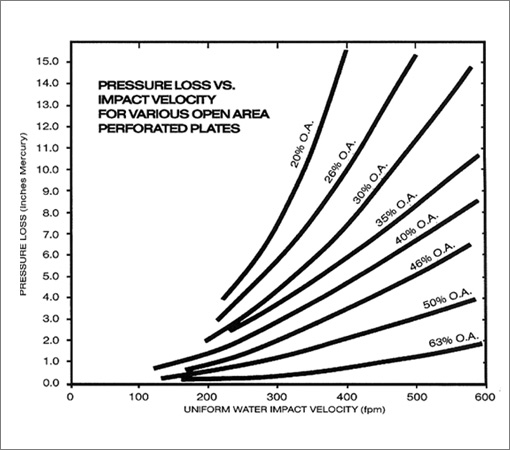# Pressure Loss

## Air Pressure Loss Through Perforated Plate

In many applications of perforated plate, the estimated energy loss or pressure loss through perforated plates is one of the design considerations. The following pressure loss information was developed from a laboratory airflow system. The laboratory system maintained a non-swirling flow impacting perpendicularly on the sample. Various perforated thin-gauge plates were inserted into a uniform velocity airflow stream. Pressure loss for ambient airflow was then measured at a series of velocities and reported as inches of water column loss for each flow.This data therefore presents the best flow condition value of loss. Pressure loss can be estimated beyond the range of the data on the basis of the ratio of the anticipated velocity to the highest tabulated velocity. This ratio squared multiplied by the tabulated pressure loss can be used to approximate the higher velocity loss.

Pressure loss can be estimated from the tables for a different gas density by using the ratio of the anticipated gas density to the tabulated density as a multiplier of the noted loss.

In applying this data, consideration must be given to the actual anticipated characteristics of the flow impacting on the perforated plate. Distorted flow patterns with high velocity zones will increase the loss of the plate, as well as directional flow not perpendicular to the plate surface.## Fluid Pressure Loss Through Perforated Plate

The following pressure loss information was developed from a laboratory liquid flow system. The laboratory system maintained a non-swirling flow impacting perpendicularly on the sample. Various perforated thin-gauge plates were inserted into a uniform velocity liquid flow stream. Pressure loss for ambient liquid flow was then measured at a series of velocities and reported as inches of mercury loss for each flow.

This data therefore presents the best flow condition value loss. Pressure loss can be estimated beyond the range of the data on the basis of the ratio of the anticipated velocity to the highest tabulated velocity. This ratio squared multiplied by the tabulated pressure loss can be used to approximate the higher velocity loss.

Pressure loss can be estimated from the tables for a different liquid density by using the ratio of the anticipated liquid density to the tabulated density as a multiplier of the noted loss.

In applying this data, consideration must be given to the actual anticipated characteristics of the flow impacting on the perforated plate. Distorted flow patterns with high velocity zones will increase the loss of the plate, as will directional flow not perpendicular to the plate surface.